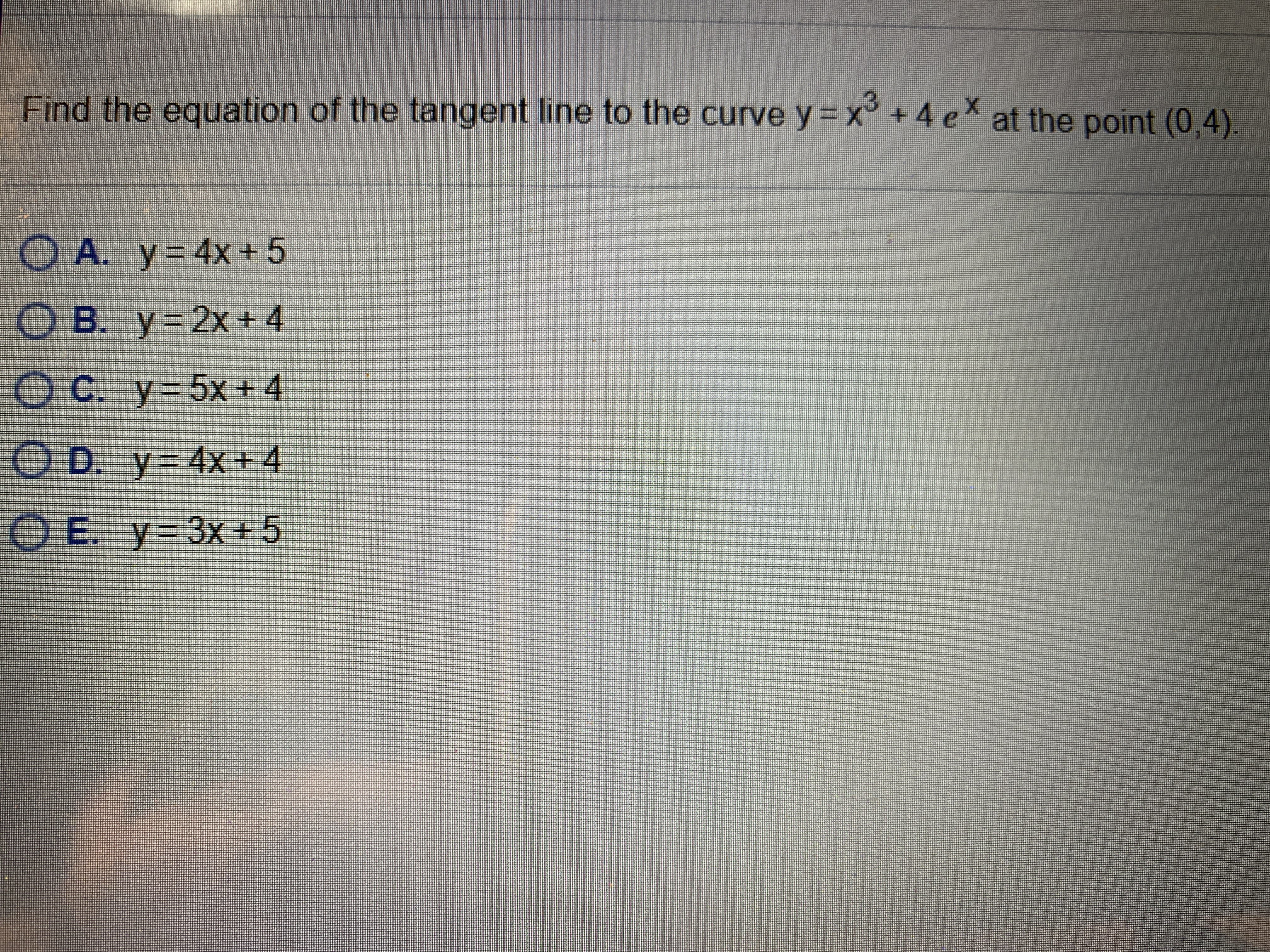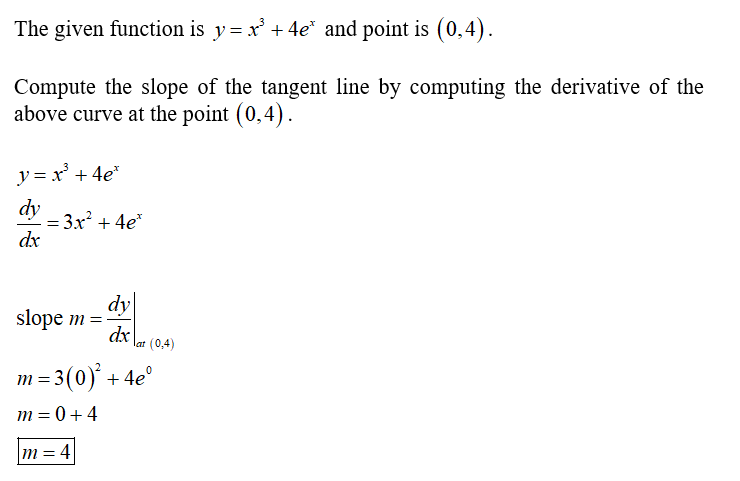# Find the equation of the tangent line to the curve y= x +4 e at the point (0,4).O A. y=4x+5O B. y=2x+4O C. y-5x+4OD. y-4X +4O E. y 3x+5

Question
1 viewshelp_outlineImage TranscriptioncloseFind the equation of the tangent line to the curve y= x +4 e at the point (0,4). O A. y=4x+5 O B. y=2x+4 O C. y-5x+4 OD. y-4X +4 O E. y 3x+5 fullscreen
check_circle

Step 1...

### Want to see the full answer?

See Solution

#### Want to see this answer and more?

Solutions are written by subject experts who are available 24/7. Questions are typically answered within 1 hour.*

See Solution
*Response times may vary by subject and question.
Tagged in